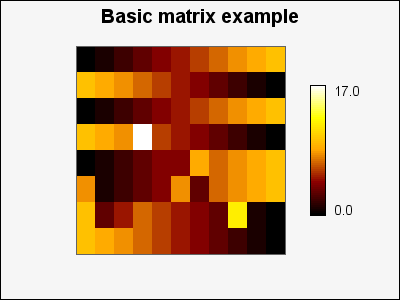## 22.2. Creating and formatting a basic matrix graph

In order to create a matrix plot the extension module "`jpgraph_matrix.php`" must be included in the script along with the core module "`jpgraph.php`".

The creation of Matrix graphs otherwise follows the traditional steps in the library of first creating a matrix graph which acts as a canvas for one or several matrix plots. The principle steps are:

1. Create a basic matrix graph as an instance of ```class MatrixGraph``` (in all our example we use the variable `\$graph` to hold this instance)

2. Create an instance of one or several matrix plots as instances of `class MatrixPlot`, make any necessary modifications to the color map and appearance and then add the plots to the matrix graph canvas.

3. Send back the graph to the client with a call to `MatrixGraph::Stroke()`. As usual this can be used to either send back the graph to the client (e.g.browser) or write the graph to a file by specifying a filename as the first argument to `MatrixGraph::Stroke()`.

The example in Figure 22.2 shows a matrix plot using just the default values for all parameters.

Example 22.1. A basic matrix graph with all default values (`matrix_ex0.php`)

 ```1 2 3 4 5 6 7 8 9 10 11 12 13 14 15 16 17 18 19 20 21 22 23 24 25 26 27 28 ``` ```title->Set('Basic matrix example'); \$graph->title->SetFont(FF_ARIAL,FS_BOLD,14); // Create a ,atrix plot using all default values \$mp = new MatrixPlot(\$data); \$graph->Add(\$mp); \$graph->Stroke(); ?>```

Figure 22.2. A basic matrix graph with all default values `(matrix_ex0.php)`In the same way as for other graph types one or several Matrix plots can be added and positioned freely in the Matrix graph by specifying the position as either absolute coordinates or as fractions of the width/height of the overall graph.

### Tip

An easier way to position Matrix plots is to use layout classes as described in Section 22.7

### Tip

If the data for the matrix is available in a file a convenient way to get hold of the dat in the file is to use the utility `class ReadFileData` to get hold of the data using the method

• `ReadFileData::FromMatrix(\$aFile,\$aSeparator=' ')`

which read the matrix from a file. Each row of the matrix must be a separate line and each cell is separated with the character specified as the second argument. By default a space is used as separator. All values read back are converted to floating point numbers (double precision). The following short example shows how easy this is to use

 ```1 ``` `\$data = ReadFileData::FromMatrix('matrixdata.txt');`

We will not further discuss all the standard graph formatting options such as the ability to add title(s), footers etc. since this is covered in numerous other places in this manual.